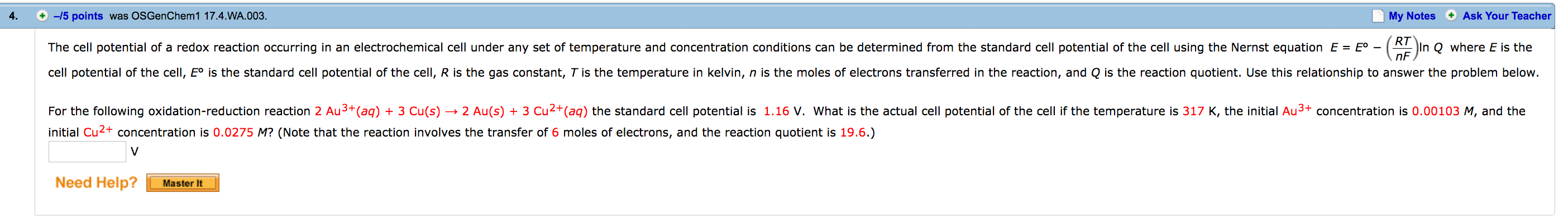# For the following oxidation-reduction reaction 2 Au3+(aq) + 3 Cu(s) + 3 Cu2+(aq) the standard potential is 1.16 V. What is the actual cell potential of the cell if the temperature is 317 K, the initial Au3+ concentration is 0.00103 M, and the initial Cu2+ concentration is 0.0275 M? (Note that the reaction involves the transfer of 6 moles of electrons, and the reaction quotient is 19.6).